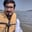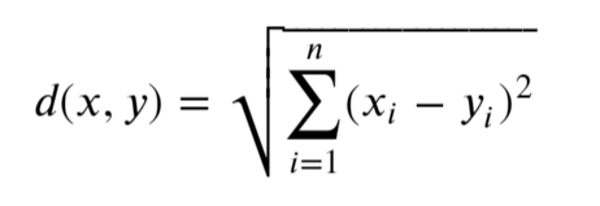Related Tags

python
numpy
euclidean
communitycreator

# How to compute the Euclidean distance between two arrays in numpyArslan Bajwa

The numpy library in Python allows us to compute Euclidean distance between two arrays.

## Euclidean distance

Euclidean distance is defined in mathematics as the magnitude or length of the line segment between two points.

## FormulaEuclidean distance formula

## Method 1

In this method, we first initialize two numpy arrays. Then, we use linalg.norm() of numpy to compute the Euclidean distance directly.

The details of the function can be found here.

#importing numpy
import numpy as np

#initializing two arrays
array1 = np.array([1,2,3,4,5])
array2 = np.array([7,6,5,4,3])

#computing the Euclidan distance
temp = array1 - array2
distance = np.linalg.norm(temp)

print("Euclidean Distance: ", distance)

## Method 2

In this method, we first initialize two numpy arrays. Then, we take the difference of the two arrays, compute the dot product of the result, and transpose of the result. Then we take the square root of the answer. This is another way to implement Euclidean distance.

#importing numpy
import numpy as np

#initializing two arrays
array1 = np.array([1,2,3,4,5])
array2 = np.array([7,6,5,4,3])

#computing the Euclidan distance
temp = array1 - array2
distance = np.sqrt(np.dot(temp.T, temp))

print("Euclidean Distance: ", distance)

## Method 3

In this method, we first initialize two numpy arrays. Then, we compute the difference of these arrays and take their square. We take the sum of the squared elements, and after that, we take the square root in the end. This is another way to implement Euclidean distance.

#importing numpy
import numpy as np

#initializing two arrays
array1 = np.array([1,2,3,4,5])
array2 = np.array([7,6,5,4,3])

#computing the Euclidan distance
temp = array1 - array2
distance = np.sqrt(np.sum(np.square(temp)))

print("Euclidean Distance: ", distance)

RELATED TAGS

python
numpy
euclidean
communitycreator

CONTRIBUTORArslan Bajwa
RELATED COURSES

View all Courses

Keep Exploring

Learn in-demand tech skills in half the time